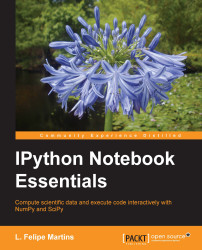•#### IPython Notebook Essentials#### Overview of this book

IPython Notebook EssentialsCreditswww.PacktPub.comPrefaceFree Chapter
A Tour of the IPython NotebookThe Notebook InterfaceGraphics with matplotlibHandling Data with pandasAdvanced Computing with SciPy, Numba, and NumbaProIPython Notebook Reference CardA Brief Review of PythonNumPy ArraysIndex## Animations

We will finish the chapter with a more complex example that illustrates the power that matplotlib gives us. We will create an animation of a forced pendulum, a well-known and much studied example of a dynamic system exhibiting deterministic chaos.

Since this section involves more sophisticated code, we will refrain from using `pylab` and adopt the generally recommended way of importing modules. This makes the code easier to export to a script if we so wish. We also give samples of some of the object-oriented features of matplotlib.

The process of animating a pendulum (or any physical process) is actually very simple: we compute the position of the pendulum at a finite number of times and display the corresponding images in quick succession. So, the code will naturally break down into the following three pieces:

• A function that displays a pendulum in an arbitrary position

• Setting up the computation of the position of the pendulum at an arbitrary time

• The code that actually computes the...